# KSEEB Solutions for Class 9 Maths Chapter 13 Surface Area and Volumes Ex 13.3

## Karnataka Board Class 9 Maths Chapter 13 Surface Area and Volumes Ex 13.3

(If values are not given for ‘n’ Assume n = $$\frac{22}{7}$$.)

Question 1.
Diameter of the base of a cone is 10.5 cm and its slant height is 10 cm. Find its curved surface area.
Solution:
Diameter of the base of a cone, d = 10.5 cm.
height h = 10 cm.
Curved Surface Area, C.S.A. = ?
d = 10.5 cm, $$=10 \frac{1}{2}=\frac{21}{2}$$ cm.
h = l = 10 cm.
∴ Curved Surface Area of a cone = πrl

Question 2.
Find the total surface area of a cone, if its slant height is 21 m and diameter of its base is 24 m.
Solution:
Diameter of base of a cone, d = 24 m.
height, h = 21 m.
Total Surface Area, T.S.A. = ?
d = 24 m, ∴ r = $$\frac{\mathrm{d}}{2}=\frac{24}{2}=12$$
h = l = 21 m.
T.S.A of a cone = πr(r + l)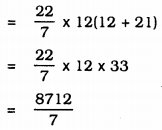= 1244.57 m2

Question 3.
Curved surface area of a cone is 308 cm2 and its slant height is 14 cm. Find
(i) radius of the base, and
(ii) total surface area of the cone.
Solution:
If Curved surface area of a cone, πrl = 308 cm2.
height, h = l = 14 cm.
Then, (i) r = ?, (ii) T.S.A. = ?
(i) CSA of a Cone = πrl∴ r = 7 cm
(ii) TSA of a cone= πr(r + l)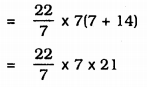= 22 × 21
= 462 cm2

Question 4.
A conical tent is 10 m high and the radius of its base is 24 m. Find
(i) slant height of the tent.
(ii) cost of the canvas required to make the tent, if the cost of 1 m2 canvas is Rs. 70.
Solution:
(i) ABC is a conical tent,
height, h = 10 m.
Slant height of the tent, l = ?In ⊥∆AOC, ∠O = 90°
As per Pythagoras theorem,
AC2 = AO2 + OC2
l2 = (01)2 +(24)2
= 100 + 576
l2 = 676
l = $$\sqrt{676}$$
Area of canvas required to prepare tent, C.S.A. = ?
C.S.A.= πrlCost of 1 m2 canvas is Rs. 70,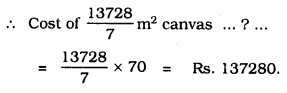Question 5.
What length of tarpaulin 3 m wide will be required to make conical tent of height 8 m and base radius 6m? Assume that the extra length of material that will be required for stitching margins and wastage in cutting is approximately 20 cm. (Use π = 3.14.)
Solution:
ABC is a conical tent.
Height, AO = h = 8 m.
base radius, OC = r = 6 cm
In ⊥∆AOC,
AC2 = AO2 + OC2
l2 = (8)2 +(6)2
l2 = 64 + 36
l2 = 100
∴ l = $$\sqrt{100}$$
l = 10 m
Curved surface Area of cone C.S.A. = πrl
= 3.14 × 6 × 10
= 188.4 m2
Let the length of tarpaulin required be 1 m.
In that 20 cm. (0.2 m) is wastage means remaining tarpaulin is (1 – 0.2 m)
∴ Area of tarpaulin = curved surface area of tent.
(l – 0.2 m) × 3 = 188.4 m2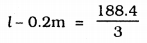l – 0.2 = 62.8
∴ l = 62.8 + 0.2
∴ l = 63 m.

Question 6.
The slant height and base diameter of a conical tomb are 25 m and 14 m respectively. Find the cost of white-washing its curved surface at the rate of Rs. 210 per 100 m2.
Solution:
Slant height of a cone, l = 25 m
Diameter, 2r = 14m ∴ r = 7 m
Curved Surface Area = πrl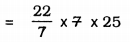= 22 × 25
= 550 m2.
Cost of white-washing is Rs. 210 per m2.
Cost of white-washing for 550 m2 … ? …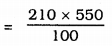= Rs. 1155.

Question 7.
A joker’s cap is in the form of a right circular cone of base radius 7 cm and height 24 cm. Find the area of the sheet required to make 10 such caps.
Solution:
A joker’s cap is in the form of a right circular cone.
radius of base, r = 7 cm.
height, h = 24 cm.
slant height, 1 = ?
Curved surface area, C.S.A.= ?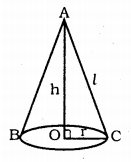In ⊥∆AOC, ∠AOC = 90°
∴ AC2 = AO2 + OC2∴ Curved surface area= πrl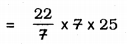= 550 m2
Area of 1 cap is 550 m2
Area of 10 caps…,v … ? …
= 550 × 10
= 5500 m2.

Question 8.
A bus stop is barricaded from the remaining part of the road, by using 50 hollow cones made of recycled cardboard. Each cone has a base diameter of 40 cm and height 1 m. If the outer side of each of the cones is to be painted and the cost of painting is Rs. 12 per m2, what will be the cost of painting all these cones ?
(Use π = 3. 14 and take $$\sqrt{1.04}$$ = 1.02)
Solution:
Diameter of a cone, d = 40 cm = 0.40 m.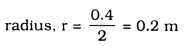height, h = l m.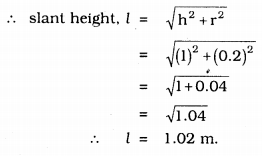Curved surface area of cone = πrl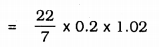= 0.64056 m2
Area of 1 cone = 0.64056 m2
Area of 50 cone = ?
= 50 × 0.64056
= 32.028 m2
Cost of painting for 1 sq.m, is Rs. 12.
Cost of painting for 32.028 sq.m. …? …
= 32.028 × 12
= Rs.384.37.

##### KSEEB Solutions for Class 9 Maths
error: Content is protected !!